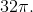# GMAT Math : DSQ: Calculating the equation of a circle

## Example Questions

### Example Question #1 : Dsq: Calculating The Equation Of A Circle

What is the equation of a given circle on the coordinate plane?

Statement 1: Its center is at the origin.

Statement 2: One of its diameters has endpoints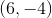and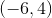.

Statement 1 ALONE is sufficient to answer the question, but Statement 2 ALONE is NOT sufficient to answer the question.

Statement 2 ALONE is sufficient to answer the question, but Statement 1 ALONE is NOT sufficient to answer the question.

BOTH statements TOGETHER are insufficient to answer the question.

EITHER statement ALONE is sufficient to answer the question.

BOTH statements TOGETHER are sufficient to answer the question, but NEITHER statement ALONE is sufficient to answer the question.

Statement 2 ALONE is sufficient to answer the question, but Statement 1 ALONE is NOT sufficient to answer the question.

Explanation:

You cannot determine the equation of a circle knowing only the center, as in Statement 1.

But given the coordinates of any diameter of a circle, as in Statement 2, you can use the midpoint formula to find the center, and the distance formula to find the distance from the center to either endpoint - this is the radius. Once you know the center and the radius, just apply them to the standard form of the equation of a circle.

### Example Question #2 : Dsq: Calculating The Equation Of A Circle

The graph of the equation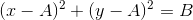,

where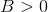, is a circle. In which quadrant is the center of the circle located?

Statement 1: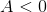Statement 2: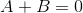Statement 1 ALONE is sufficient to answer the question, but Statement 2 ALONE is NOT sufficient to answer the question.

BOTH statements TOGETHER are insufficient to answer the question.

Statement 2 ALONE is sufficient to answer the question, but Statement 1 ALONE is NOT sufficient to answer the question.

EITHER statement ALONE is sufficient to answer the question.

BOTH statements TOGETHER are sufficient to answer the question, but NEITHER statement ALONE is sufficient to answer the question.

EITHER statement ALONE is sufficient to answer the question.

Explanation:

The center of the circle of the equationis the point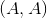.

If Statement 2 is assumed, then; sinceis known to be positive,is negative, which is the same as Statement 1.

From either statement, we knowto be negative, and, subsequently, that both coordinates of the centerare negative, putting it in Quadrant III.

### Example Question #51 : Coordinate Geometry

Find the equation of circle T.

I) Circle T is centered at the point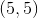.

II) Circle T touches theaxis at the point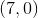.

Each statement alone is enough to solve the question.

Statement 2 is sufficient to solve the question, but statement 1 is not sufficient to solve the question.

Both statements taken together are sufficient to solve the question.

Neither statement is sufficient to solve the question. More information is needed.

Statement 1 is sufficient to solve the question, but statement 2 is not sufficient to solve the question.

Both statements taken together are sufficient to solve the question.

Explanation:

The equation of a circle is given by: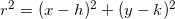Where r is the radius and (h,k) are the center of the circle

I) gives us (h,k)

By using II) and distance formula, we can find r

Thus, both statements are needed!

### Example Question #4 : Dsq: Calculating The Equation Of A Circle

Write the equation for circle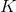, a circle in the standard x-y plane.

I)is centered at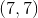.

II) The line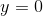is tangent to the circle at the point.

Both statements together are needed to answer the question.

Statement II is sufficient to answer the question, but Statement I is not sufficient to answer the question.

Either statement alone is sufficient to answer the question.

Statement I is sufficient to answer the question, but Statement II is not sufficient to answer the question.

Both statements together are needed to answer the question.

Explanation:

To write the equation of a circle we need the coordinates of its center and the length of its radius.

I) Gives us the center.

II) Tells us that the circle just touches the x-axis at the point (7,0), which is exactly 7 units below our center. This makes our radius 7.

Thus, we need both statements.

### Example Question #5 : Dsq: Calculating The Equation Of A Circle

Consider Circle L.

I) The radius of Circle L is twice the value of the third prime number when counting the prime numbers and increasing after zero.

II) The x-coordinate of Circle L is four times the y-coordinate of L. The y-coordinate is equal to the radius.

Find the equation of Circle L.

Statement II is sufficient to answer the question, but Statement I is not sufficient to answer the question.

Either statement is sufficient to answer the question.

Statement I is sufficient to answer the question, but Statement II is not sufficient to answer the question.

Both statements are needed to answer the question.

Both statements are needed to answer the question.

Explanation:

The equation of a circle is as follows: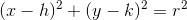Where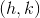is the center of the circle andis the radius.

Statement I gives us the radius.

Statement II gives us clues to find.

So, by using both, we can find the equation. Both are needed.

Recap:

Consider circle L.

I) The radius of L is twice the value of the third prime number.

II) The x-coordinate of L is four times the y-coordinate of L. The y-coordinate is equal to the radius.

Find the equation of circle L.

Using Statement I, the third prime number is 5, so our radius is 10.

Using Statement II,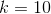, somust be 40.

Putting it all into the equation of a circle: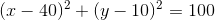### Example Question #6 : Dsq: Calculating The Equation Of A Circle

Find the equation of Circle X.

I) The x-coordinate of Circle X's center is twice the y-coordinate of Circle X's center.

II) Circle X has a radius of 16 units.

Both statements are needed to answer the question.

Statement II is sufficient to answer the question, but Statement I is not sufficient to answer the question.

Either statement is sufficient to answer the question.

Statement I is sufficient to answer the question, but Statement II is not sufficient to answer the question.

Explanation:

The equation of a circle is as follows:Whereis the center of the circle andis the radius.

Statement I tells us how to relateand, but doesn't tell us how to find either one.

Statement II gives us.

We almost have enough information here, but we can't tell for certain where the circle's center is, so more information is needed.

### Example Question #7 : Dsq: Calculating The Equation Of A Circle

Circle T is plotted in Euclidean space. Find its equation.

I) The diameter of Circle T is twice the square of the y-coordinate of its center.

II) Circle T is centered at the point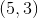.

Either statement is sufficient to answer the question.

Statement II is sufficient to answer the question, but Statement I is not sufficient to answer the question.

Statement I is sufficient to answer the question, but Statement II is not sufficient to answer the question.

Both statements are needed to answer the question.

Both statements are needed to answer the question.

Explanation:

The equation of a circle is as follows:Whereis the center of the circle andis the radius.

Statement I gives us a clue to find the diameter, which can be used to find the radius.

Statement II gives us the center of the circle.

Use Statement II and Statement I to find the radius, then plug it all into the equation to find the equation of Circle T.

Recap:

Circle T is plotted in Euclidean space. Find its equation.

I) The diameter of circle T is twice the square of the y-coordinate of its center.

II) Circle T is centered at the point.

Statement II gives us.

Use Statement I and Statement II to write the following equation: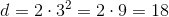So, if the diameter is 18, the radius must be 9. This makes our equation of the circle: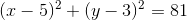### Example Question #8 : Dsq: Calculating The Equation Of A Circle

What is the area of the circle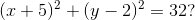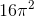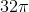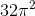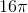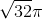Circle equations are written in the form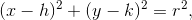whereare the coordinates for the center of the circle.  When dealing with the area of a circle, the formula is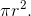Since, the circle equation already gives us the squared radius, we only need to multiply that number by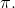Therefore, the area is